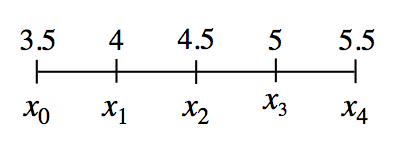### Home > PC3 > Chapter 3 > Lesson 3.2.4 > Problem3-135

3-135.Consider the values on the $x$-axis and their corresponding subscript values as shown at right.

1. Write a linear equation for $x_k$.

Write the equation of a linear function using the points $(0,3.5)$ and $(1,4)$.

2. Express $3.5+4+4.5+5+5.5$ in sigma notation.

Use your answer to part (a), making sure your stating index is $0$.

3. For some function, $f$, express $f(3.5)+f(4)+f(4.5)+f(5)+f(5.5)$ in sigma notation.

This is similar to your answer to part (b), but using function notation.

4. How are parts (a), (b), and (c) related?